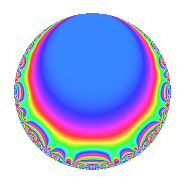# Properties

 Label 123.2.a.cLevel 123 Weight 2 Character orbit 123.a Self dual Yes Analytic conductor 0.982 Analytic rank 0 Dimension 2 CM No Inner twists 1

# Related objects

## Newspace parameters

 Level: $$N$$ = $$123 = 3 \cdot 41$$ Weight: $$k$$ = $$2$$ Character orbit: $$[\chi]$$ = 123.a (trivial)

## Newform invariants

 Self dual: Yes Analytic conductor: $$0.982159944862$$ Analytic rank: $$0$$ Dimension: $$2$$ Coefficient field: $$\Q(\sqrt{2})$$ Coefficient ring: $$\Z[a_1, a_2]$$ Coefficient ring index: $$1$$ Fricke sign: $$-1$$ Sato-Tate group: $\mathrm{SU}(2)$

## $q$-expansion

Coefficients of the $$q$$-expansion are expressed in terms of $$\beta = \sqrt{2}$$. We also show the integral $$q$$-expansion of the trace form.

 $$f(q)$$ $$=$$ $$q + \beta q^{2} + q^{3} + ( 2 - \beta ) q^{5} + \beta q^{6} + ( -2 + \beta ) q^{7} -2 \beta q^{8} + q^{9} +O(q^{10})$$ $$q + \beta q^{2} + q^{3} + ( 2 - \beta ) q^{5} + \beta q^{6} + ( -2 + \beta ) q^{7} -2 \beta q^{8} + q^{9} + ( -2 + 2 \beta ) q^{10} + ( 1 - \beta ) q^{11} + ( 2 - 3 \beta ) q^{13} + ( 2 - 2 \beta ) q^{14} + ( 2 - \beta ) q^{15} -4 q^{16} + ( 1 + \beta ) q^{17} + \beta q^{18} + ( -4 + \beta ) q^{19} + ( -2 + \beta ) q^{21} + ( -2 + \beta ) q^{22} + \beta q^{23} -2 \beta q^{24} + ( 1 - 4 \beta ) q^{25} + ( -6 + 2 \beta ) q^{26} + q^{27} + ( 1 + 5 \beta ) q^{29} + ( -2 + 2 \beta ) q^{30} -3 q^{31} + ( 1 - \beta ) q^{33} + ( 2 + \beta ) q^{34} + ( -6 + 4 \beta ) q^{35} + ( -1 + 6 \beta ) q^{37} + ( 2 - 4 \beta ) q^{38} + ( 2 - 3 \beta ) q^{39} + ( 4 - 4 \beta ) q^{40} - q^{41} + ( 2 - 2 \beta ) q^{42} -5 q^{43} + ( 2 - \beta ) q^{45} + 2 q^{46} + ( 9 - \beta ) q^{47} -4 q^{48} + ( -1 - 4 \beta ) q^{49} + ( -8 + \beta ) q^{50} + ( 1 + \beta ) q^{51} + ( 4 - 2 \beta ) q^{53} + \beta q^{54} + ( 4 - 3 \beta ) q^{55} + ( -4 + 4 \beta ) q^{56} + ( -4 + \beta ) q^{57} + ( 10 + \beta ) q^{58} + 6 \beta q^{59} + ( 1 + 4 \beta ) q^{61} -3 \beta q^{62} + ( -2 + \beta ) q^{63} + 8 q^{64} + ( 10 - 8 \beta ) q^{65} + ( -2 + \beta ) q^{66} + ( 2 - 6 \beta ) q^{67} + \beta q^{69} + ( 8 - 6 \beta ) q^{70} + ( 3 - 5 \beta ) q^{71} -2 \beta q^{72} + ( 1 + 8 \beta ) q^{73} + ( 12 - \beta ) q^{74} + ( 1 - 4 \beta ) q^{75} + ( -4 + 3 \beta ) q^{77} + ( -6 + 2 \beta ) q^{78} + ( -2 + 4 \beta ) q^{79} + ( -8 + 4 \beta ) q^{80} + q^{81} -\beta q^{82} + ( -6 - 5 \beta ) q^{83} + \beta q^{85} -5 \beta q^{86} + ( 1 + 5 \beta ) q^{87} + ( 4 - 2 \beta ) q^{88} + ( -6 + 4 \beta ) q^{89} + ( -2 + 2 \beta ) q^{90} + ( -10 + 8 \beta ) q^{91} -3 q^{93} + ( -2 + 9 \beta ) q^{94} + ( -10 + 6 \beta ) q^{95} + ( 12 + 3 \beta ) q^{97} + ( -8 - \beta ) q^{98} + ( 1 - \beta ) q^{99} +O(q^{100})$$ $$\operatorname{Tr}(f)(q)$$ $$=$$ $$2q + 2q^{3} + 4q^{5} - 4q^{7} + 2q^{9} + O(q^{10})$$ $$2q + 2q^{3} + 4q^{5} - 4q^{7} + 2q^{9} - 4q^{10} + 2q^{11} + 4q^{13} + 4q^{14} + 4q^{15} - 8q^{16} + 2q^{17} - 8q^{19} - 4q^{21} - 4q^{22} + 2q^{25} - 12q^{26} + 2q^{27} + 2q^{29} - 4q^{30} - 6q^{31} + 2q^{33} + 4q^{34} - 12q^{35} - 2q^{37} + 4q^{38} + 4q^{39} + 8q^{40} - 2q^{41} + 4q^{42} - 10q^{43} + 4q^{45} + 4q^{46} + 18q^{47} - 8q^{48} - 2q^{49} - 16q^{50} + 2q^{51} + 8q^{53} + 8q^{55} - 8q^{56} - 8q^{57} + 20q^{58} + 2q^{61} - 4q^{63} + 16q^{64} + 20q^{65} - 4q^{66} + 4q^{67} + 16q^{70} + 6q^{71} + 2q^{73} + 24q^{74} + 2q^{75} - 8q^{77} - 12q^{78} - 4q^{79} - 16q^{80} + 2q^{81} - 12q^{83} + 2q^{87} + 8q^{88} - 12q^{89} - 4q^{90} - 20q^{91} - 6q^{93} - 4q^{94} - 20q^{95} + 24q^{97} - 16q^{98} + 2q^{99} + O(q^{100})$$

## Embeddings

For each embedding $$\iota_m$$ of the coefficient field, the values $$\iota_m(a_n)$$ are shown below.

For more information on an embedded modular form you can click on its label.

Label $$\iota_m(\nu)$$ $$a_{2}$$ $$a_{3}$$ $$a_{4}$$ $$a_{5}$$ $$a_{6}$$ $$a_{7}$$ $$a_{8}$$ $$a_{9}$$ $$a_{10}$$
1.1
 −1.41421 1.41421
−1.41421 1.00000 0 3.41421 −1.41421 −3.41421 2.82843 1.00000 −4.82843
1.2 1.41421 1.00000 0 0.585786 1.41421 −0.585786 −2.82843 1.00000 0.828427
 $$n$$: e.g. 2-40 or 990-1000 Significant digits: Format: Complex embeddings Normalized embeddings Satake parameters Satake angles

## Inner twists

This newform does not admit any (nontrivial) inner twists.

## Atkin-Lehner signs

$$p$$ Sign
$$3$$ $$-1$$
$$41$$ $$1$$

## Hecke kernels

This newform can be constructed as the kernel of the linear operator $$T_{2}^{2} - 2$$ acting on $$S_{2}^{\mathrm{new}}(\Gamma_0(123))$$.